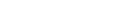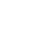Scalar Operators

Numerical operators

The types int, long, and real represent numerical types. The following operators can be used between pairs of these types:

OperatorDescriptionExample
+Add3.19 + 3.19, ago(10m) + 10m
-Subtract0.26 - 0.23
*Multiply1s * 5, 5 * 5
/Divide10m / 1s, 4 / 2
%Modulo10 % 3, 5 % 2
<Less1 < 2, 1 <= 1
>Greater0.23 > 0.22, 10min > 1sec, now() > ago(1d)
==Equals3 == 3
!=Not equals2 != 1
<=Less or Equal5 <= 6
>=Greater or Equal7 >= 6
inEquals to one of the elements"abc" in ("123", "345", "abc")
!inNot equals to any of the elements"bca" !in ("123", "345", "abc")Unable to retrieve cloud status